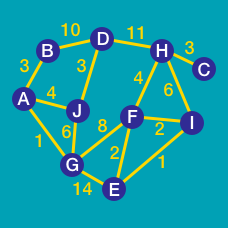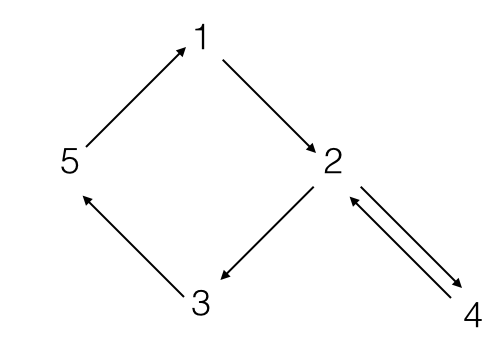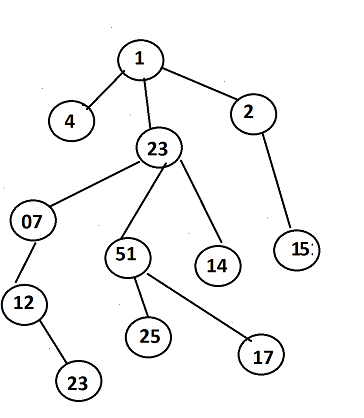Computer Science

# Graph Algorithms

#### Challenge Quizzes

Use the breadth first strategy to traverse the graph belowList the vertices in the order in which breadth first search traverses them.

• Start from the node $1$.
• With the choice of two children, visit first the one of higher value.

Given a complete binary tree $T$ with $n$ nodes, which of the following best describes the time it takes to find a path using breadth first search from a root $V$ to another node $s$ ($s \in T$)?Consider the above tree. Using breadth first search, we can trace the path $P$ from any node $S$ to any other node $V$. For example the path from $S=1$ to $V=15$ is $\{1,2,15\}$.

Let us define a function $sP(S,V)$ that returns the sum of the nodes in the path $P$. So for $S=1$ and $V=15$, $sP(1,15)=sum(\{1,2,15\})=18$.

Find the value of $\sum_{i=1}^{12} sP(1,v_i)$ where $v_i$ is the $i$th node in the tree and $v_i \neq 1$.

×